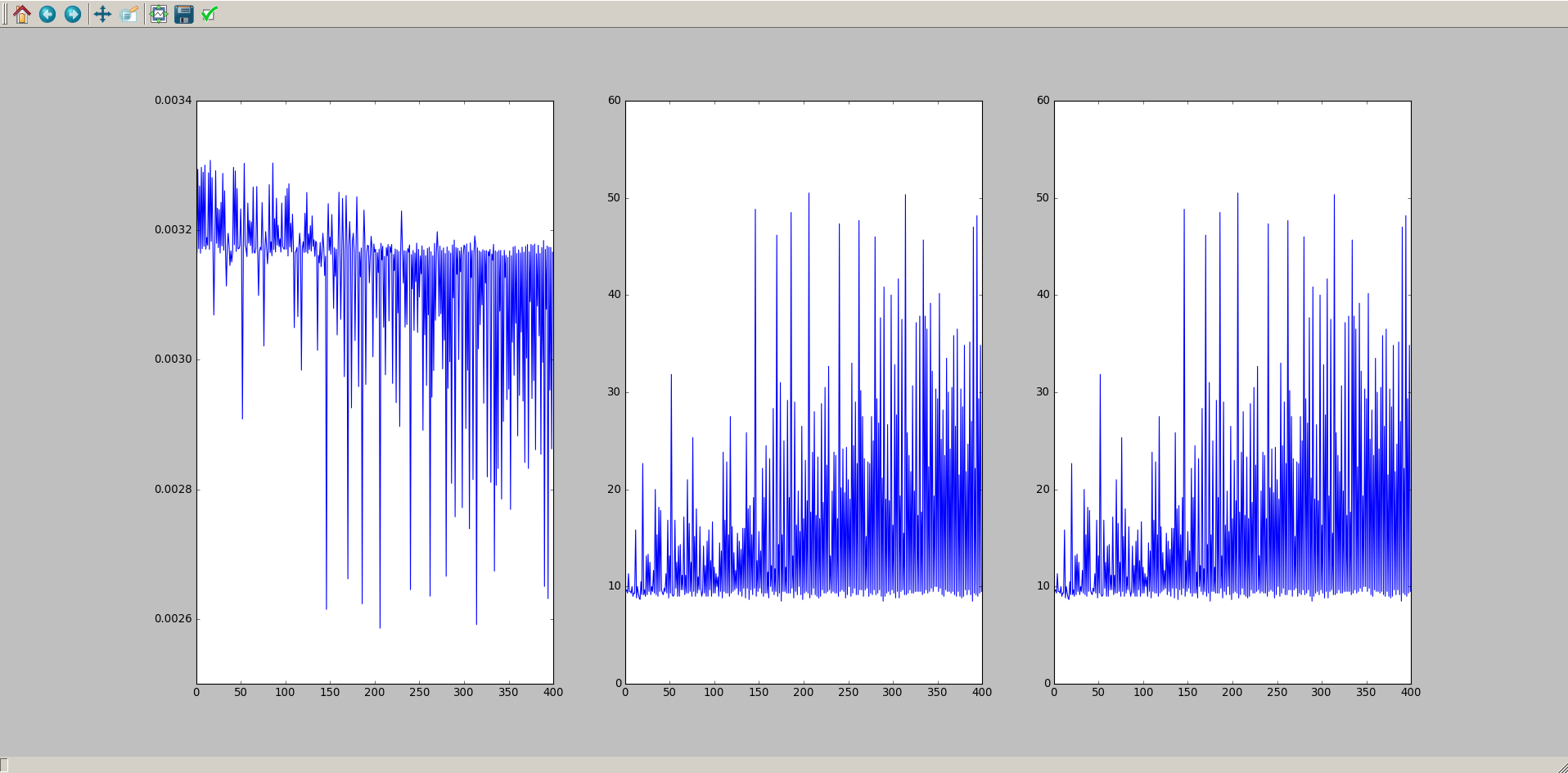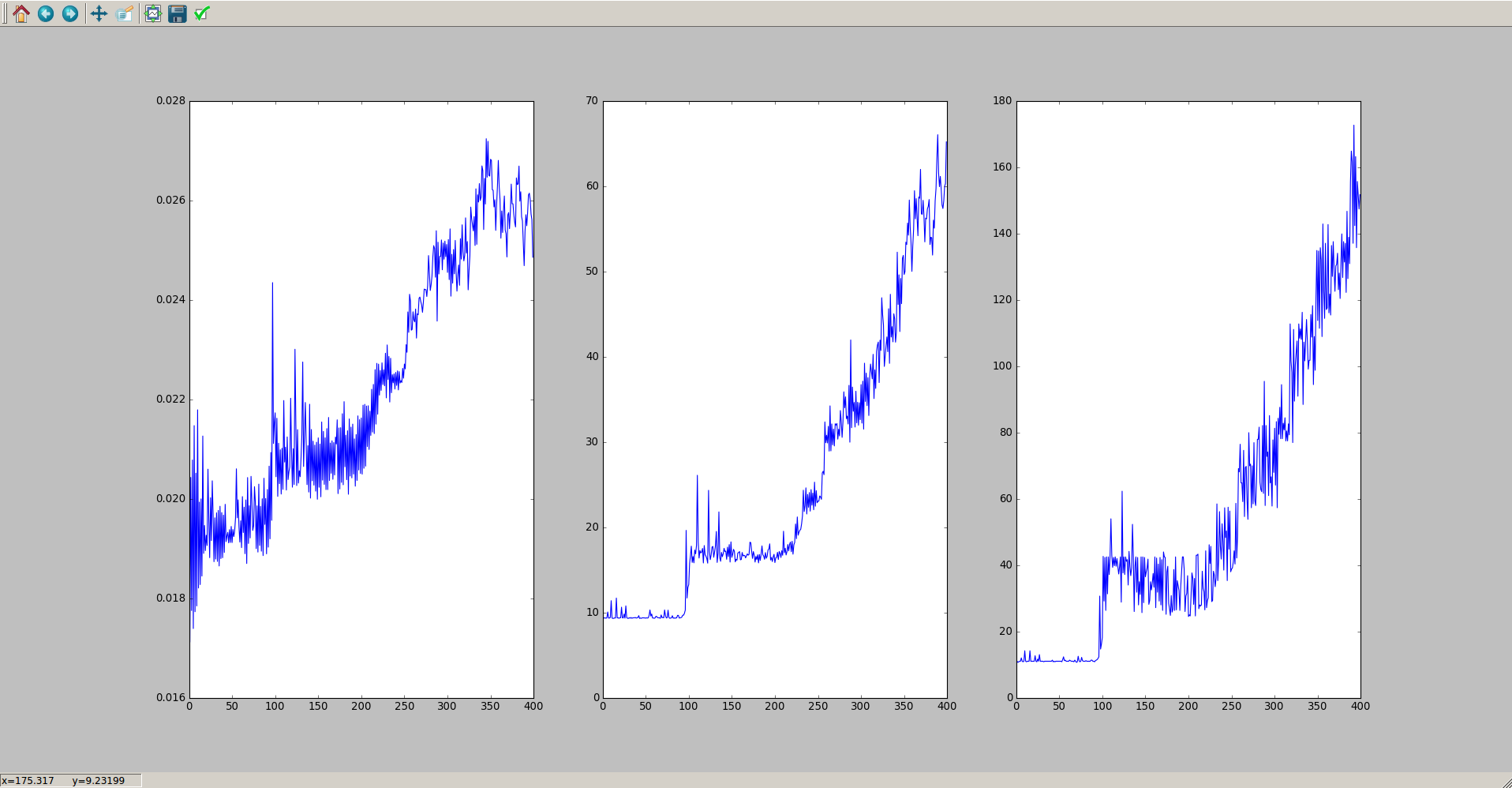# Bayesian Optimization of Black Box Functions – Appendix and Sources / Resources

## Examples of the Power of Bayesian Black Box Optimization

### 1. 10 Evaluations of a sample Black Box Function$e^{-(x-2)^2} + e^{\frac{-(x-6)^2}{10}} + \frac{1}{x^2 + 1}$Best input found: 2, Global Maximum: 2

### 2. Policy Gradient Reinforcement Learning

For this one, I used the hyperparameters for my policy gradient learner as inputs to the function. With the mini-batch size, learning rate, learning rate exponential decay rate, and discount factor, it had four different inputs to tune, with a level of detail of just 10 for each input. It ran each input three times and averaged the output to increase reliability, and each run took from one to five minutes.

I used the cartpole reinforcement learning environment provided by OpenAI, ran for 400 epochs, with 200 timesteps. I used the Matern covariance function (credit to Jasper Snoek, Hugo Larochelle and Ryan P. Adams from various universities for their paper in which they detail this covariance function), and the Upper confidence bound covariance function with confidence interval of 1.5.

It used a mini batch size range of 1 to 100, learning rate range of 1 to 10, learning rate decay rate range of 0 to 10 (where the value is plugged into -x/epochs, I have found this tends to give nice exponential decay curves), and discount factor range of 0 to 1.

For this comparison of methods, I did the following for each. I averaged over six runs. The subplots represent cost after each epoch, average timesteps survived over each mini batch after each epoch, and max timesteps survived over each mini batch after each epoch

I ran my grid search alternative algorithm on it for as long as it wanted, which was an excess of 200 evaluations. With that, it obtained the following results with the final parameters:I then ran my bayesian optimization bot on it for 20 evaluations, and obtained the following:So we can see that in a tenth of the evaluations, Bayesian Optimization won out by a longshot, and achieved parameters good enough to beat the cartpole environment on OpenAI.

## More Covariance Functions$For\ two\ inputs\ x_i,\ x_j\ and\ free\ parameters\ \alpha\ and\ \beta$

### 1. Dot Product$cov(x_i, x_j) = \alpha \left( x_i^T \cdot x_j \right)$

### 2. Brownian Motion$cov(x_i, x_j) = \alpha min(x_i, x_j)$

### 3. Squared Exponential$cov(x_i, x_j) = e^{-\alpha ||x_i - x_j||^2}$

### 4. Ornstein-Uhlenbeck$cov(x_i, x_j) = e^{-\alpha \sqrt{||x_i - x_j||^2}}$

### 5. Example Periodic Function$cov(x_i, x_j) = e^{-\alpha \sin(\beta \pi ||x_i - x_j||^2}$

### 6. Matérn Covariance Function*$d = ||x_i - x_j||^2 \\ cov(d) = \left(1 + \sqrt{5d} + \frac{5}{3}d \right) e^{-\sqrt{5d}}$

* = Used this for cartpole test and has been best for me so far

## More Acquisition Functions$For\ test\ mean\ \mu_*\ and\ test\ variance\ \sigma_*^2$

### 1. Upper Confidence Bound*$= argmax \left( \mu_* + \kappa \sigma_*^2 \right)$

### 2. Probability of Improvement**$= argmax \left( \Phi \left( f_*; \mu_*, \sigma_*^2 \right) \right)$

### 3. Expected Improvement$= argmax \left( \sigma_* \mathcal{N}(f_*;\mu_*,\sigma_*) \Phi \left( f_*; \mu_*, \sigma_*^2 \right) \mathcal{N}(f_*;0, 1) \right)$

* – Used this for cartpole test and has been best for me so far with$\kappa = 1.5$ , also keep in mind this is the form for a maximization problem (e.g. accuracy), however the standard deviation term would be negative for a minimization problem (e.g. cost).

** –$\Phi$ is the cumulative distribution function up to the point given.$f_*$ is our$\mu_* \pm \sigma_*^2$

Resources & Sources

### Videos and Video Lectures

• Several videos from mathematicalmonk on youtube, from whence I learned the first five of these covariance functions and about Unconstrained Gaussian Processes.
• A couple videos from the University of British Columbia’s Computer Science youtube lectures, from whence I learned about Constrained Gaussian Processes, including the necessary covariance matrices/vectors and the Multivariate Gaussian Distribution theorems. Go here to learn all about the math behind constraining Gaussian Processes for regression.

### Presentation Slides and Miscellaneous

• A Tutorial on Gaussian Processes, By Zoubin Ghahramani of the University of Cambridge(UK). I learned more about different free parameters in covariance functions such as lengthscales from these slides, though they also give a more intermediate tutorial on Gaussian Processes.
• Gaussian ProcessesBy Daniel McDuff of MIT. Good information on Covariance functions, covariance, and Gaussian Process regression again.
• A Tutorial on Bayesian Optimization for Machine LearningBy Ryan P. Adams of Harvard University. This gives a great visualization of different acquisition functions, how we use Gaussian Processes for Bayesian Optimization, as well as different types of covariance functions. It also speaks on a variant of the Matérn Covariance Function that I couldn’t get working, and integrating out Gaussian Process Hyper Parameters, which I didn’t implement.
• fernando on Github’s Bayesian Optimization Visualization iPython Notebook, whom had a really nice test black box function which I used at the top of this appendix.
• Guassian Random Vectors, By Robert G. Gallager of MIT. Go here for anything and everything about Gaussian Random Vectors, with a lot of math (you’ve been warned).
• Regression, By Robert G. Gallager of MIT. Go here for anything and everything about Gaussian Regression, with a lot of math (you’ve been warned).
• Covariance Functions, By Robert G. Gallager of MIT. Go here for anything and everything about covariance functions, with a lot of math (you’ve been warned).
• Gaussian Processes Covariance Functions and ClassificationBy Carl Edward Rasmussen of the Max Planck Institute for Biological Cybernetics. This is another overarching slide run-through.
• CSE515 Lecture Notes, By Roman Garnett of Washington University in St. Louis. This quickly and succinctly covers the most common acquisition functions, and I learned them first from here.

You can use any of my stuff (but it wouldn’t hurt to remember my name), and everything I’ve written in this series comes from these sources, or from my code that is openly available on my Github. Good luck, have fun.

-Dark Element